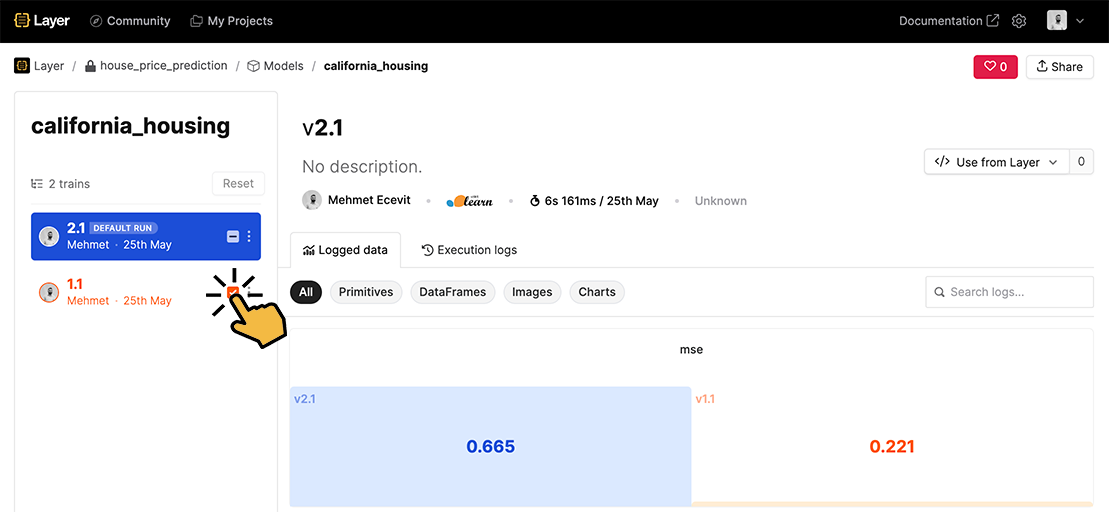# How to register and track your model with Layer

Layer helps you build, train and track all your machine learning project metadata including ML models and datasets‍ with semantic versioning, extensive artifact logging and dynamic reporting with local↔cloud training.

In this quick walkthrough, we'll take a look at how to register and track ML models with Layer.

## Installation​

``!pip install -U layer -q!pip install -U xgboost==1.5.1!pip install -U ipython``
``from sklearn.datasets import fetch_california_housingfrom sklearn.metrics import mean_squared_errorfrom sklearn.model_selection import train_test_splitfrom layer.decorators import modelimport seaborn as snsimport xgboost as xgbimport pandas as pdimport layer``

## Inititialize Your First Layer Project​

It's time to create your first Layer project!

Layer Projects are smart containers to organize your machine learning metadata such as models, datasets, metrics, reports etc. They associate some combination of datasets and models. Layer projects are basically the front page of your ML projects which includes all your ML metadata including ML models, datasets, metric, parameters and more.

``# Log in to your free account!layer.login()``
``layer.init("house_price_prediction")``

## Train and Register Your Model​

In this tutorial, we will be training a `XGBRegressor` to predict the house prices. We can see that this is a simple training function which returns the trained model and prints the `mean_square_error` of our model. We are going to use the `california_housing` data from `sklearn`.

``X, y = fetch_california_housing(return_X_y=True)X_train, X_test, y_train, y_test = train_test_split(X, y, random_state=0)``
``def train():    xgb_model = xgb.XGBRegressor()    xgb_model.fit(X_train, y_train)        predictions = xgb_model.predict(X_test)    mse = mean_squared_error(y_test, predictions)        print("mse: ", mse)        return xgb_modelxgb_model = train()``

### 🔥 Now let's register our model to Layer!​

Simply add the `@model` decorator to your training function. Layer will register the returned trained model to your Layer Project. Let's also replace `print()` with `layer.log()` to enable experiment tracking.

``@model("california_housing")def train():    xgb_model = xgb.XGBRegressor()    xgb_model.fit(X_train, y_train)        predictions = xgb_model.predict(X_test)    mse = mean_squared_error(y_test, predictions)        layer.log({"mse": mse})        return xgb_modelxgb_model = train()``

⬆️ Click the above link to see the registered model in your Layer Project. You will see that Layer automatically registered and versioned your model.

Let's do some manual hyperparameter tuning and compare our experiments if it changed anything on the `mse` metric.

``@model("california_housing")def train():    xgb_model = xgb.XGBRegressor(max_depth=parameters["max_depth"], n_estimators=parameters["n_estimators"])    xgb_model.fit(X_train, y_train)    predictions = xgb_model.predict(X_test)        mse = mean_squared_error(y_test, predictions)        layer.log(parameters)    layer.log({"mse": mse})        return xgb_modelparameters = {"max_depth":1, "n_estimators":10}xgb_model = train()``

⬆️ Click the above link to compare your `mse` metric between your two training. Just click the checkbox by your first model version as shown in the image. Layer will show you how your `mse` metric has changed between these runs.Now let's test different parameters. By the way, you can log plots and tables with `layer.log` too.

``@model("california_housing")def train():    xgb_model = xgb.XGBRegressor(max_depth=parameters["max_depth"], n_estimators=parameters["n_estimators"])    xgb_model.fit(X_train, y_train)        predictions = xgb_model.predict(X_test)        mse = mean_squared_error(y_test, predictions)        table = pd.DataFrame(zip(predictions,y_test),columns=['Predicted Price', "Actual Price"])    plot = sns.histplot((y_test-predictions), kde=True).figure    layer.log({"Predictions":plot})    layer.log({"Predictions vs Actual":table[:50]})    layer.log(parameters)    layer.log({"mse": mse})        return xgb_modelparameters = {"max_depth":4, "n_estimators":100}xgb_model = train()``

⬆️ Clicking the above link you will find the logged tables and plots. You can do comparison with multiple versions of your models. Try it now!

### 🌐 Remote Training 🌐​

Layer is a very advanced metadata store where you can store your models, datasets but also your pipelines. Just like you registered your model, you can also register and execute your machine learning pipeline on Layer. This is especially usefull when:

• Your training data is too big too fit in your local machine.
• Your model requires special infra like a high-end GPU that you don't have locally.

Now instead of running your `train` function directly, just pass it to Layer with `layer.run([train])`. Layer will pickle your function and run it on Layer infra.

``layer.run([train])``

Once you register your model to Layer, you can load your model from Layer with simple calling `layer.get_model(MODEL_NAME)`
``xgb_model = layer.get_model("california_housing").get_train()X, y = fetch_california_housing(return_X_y=True)preds = xgb_model.predict(X)print("Prediction: ", preds)``